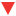IT-technology## 香农定理与奈奎斯特定理问题：

### 香农定理

``Vmax=W log2(1+S/N)  单位(b/s)``

``dB=10log10S/N     dB的单位为 分贝``

• 根据题意例出香农定理算式：Vmax=Wlog2(1+S/N)
• 例出信噪比关系：dB=10log10S/N
• 计算 30dB=10log10S/N 则S/N=1000
• Vmax=4Khz log2(1+1000)=4000x10 =40000b/s=40kb/s

### 尼奎斯特定理

``B=2W（baud）``

``n=log2N``1391-024-6332